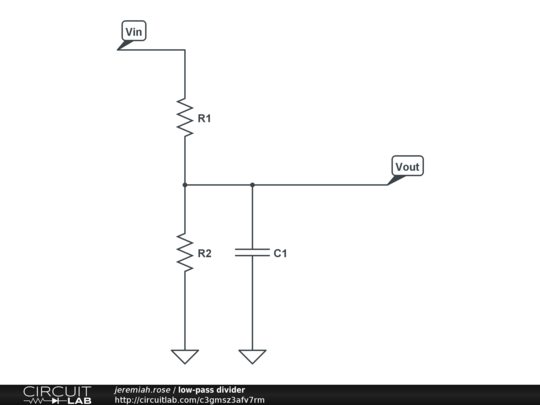# Divider and lowpass combined

I am using the following circuit for two purposes:

• As a voltage divider, to reduce DC sensor output to a range suitable for my ADC.
• As a lowpass filter, to remove high frequency noise from the signal.It works great, but my choice of component values has largely been a matter of trial and error, so I have a few questions:

1. What is this type of circuit called? Is it a low-pass filter, a voltage divider or both?
2. What is the equation for $f_c$ (cutoff frequency) for this circuit and how is it derived?
3. What is the output impedance $Z_{out}$ of the circuit in terms of $R_1$, $R_2$ and $C_1$?
4. If the design requires a specific $f_c$, $Z_{out}$ and DC $\frac{V_{out}}{V_{in}}$, is it possible to derive simple equations for $R_1$, $R_2$ and $C_1$ in terms of these?

1. Not that I'm aware of, call it a filtered voltage divider :)

2. $$\fc=\frac{(R_1+R_2)\sqrt{(10^{3/10}-1)}}{2\pi*R_1*R_2*C_1}\approx \frac{(R_1+R_2)}{2\pi*R_1*R_2*C_1} \$$

3. $$\R_1//R_2//C_1 \$$

4. There are other considerations to take into account. In your case for instance, the conversion speed (the time it takes to charge the ADC's internal sampling capacitor) which would have an impact on the max series resistance, max Vin voltage and so on.

$R_1 = \frac{V_{in} R_{out}}{V_{out}}$

$R_2 = \frac{V_{in} R_{out}}{V_{in} - V_{out}}$

$C_1 = \frac{1}{2 \pi f_c R_{out}}$

Where $R_{out}$ is the DC output impedance ($\omega = 0$) and $f_c$ is the low-pass cutoff frequency.

1) I call this a voltage divider with a filter cap. There's no special name for it as far as I know

2) $f_c = \frac{1}{2\pi * (R_1//R_2) * C_1}$

Where $R_1//R_2$ is the value you get when $R_1$ and $R_2$ are in parallel

3) can be answered by learning some basic network theory. This is explained in any textbook about electronics or electronic networks.

4) yes, by learning what is mentioned under 3)